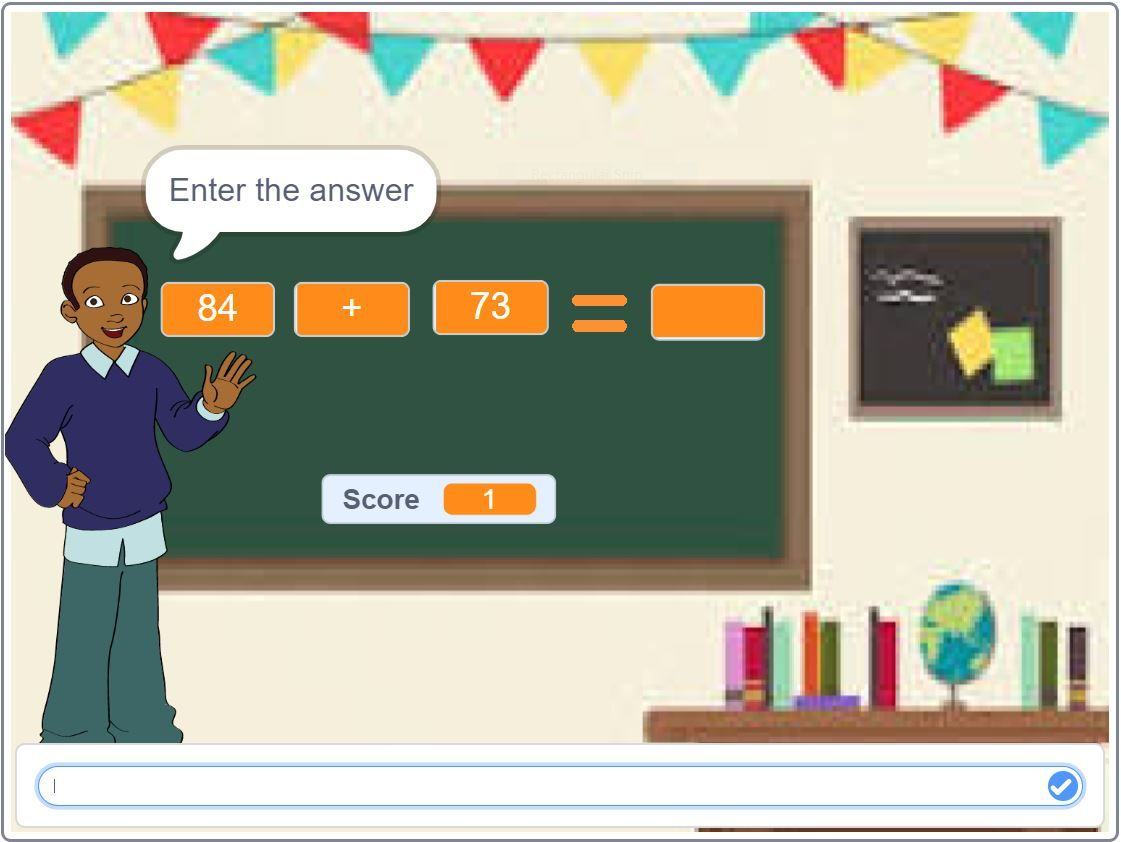# Mathematics and Coding: The Perfect Partnership for Problem-Solving and Logical ThinkingDo you enjoy puzzles and problem-solving? If so, you’ll love mathematics and coding! These two disciplines are perfect for logical thinkers who want a challenge. Mathematics is all about solving problems with precision and structure. Coding is the process of transforming written instructions into a form that a computer can understand. When these two fields are combined, they create a powerful toolset for solving complex problems. In this blog post, we will explore the ways in which mathematics and coding intersect, and why it’s so important for kids to learn both subjects.

Both of these subjects require two basic steps: Understanding the problem and persevering in solving it. Eventually, every coding problem is an underlying mathematics problem where coding has been used simply as a tool to perform computation and obtain the output.

Here are some of the skills that are common in both Mathematics and Programming:

## Problem-Solving

In order to write code, you need to be able to think logically and break down problems into smaller, manageable pieces. This is a skill that is essential for success in mathematics. In both mathematics and coding, it is often helpful to approach a problem from multiple angles. By looking at a problem from different perspectives, you can gain new insights and find more efficient solutions.

## Logical Thinking

One of the fundamental skills required to excel in mathematics is logic. Mathematicians use inference and reasoning to find solutions.

Similarly, in order to build a new app, game, or cartoon the programmer must think logically about what the character in the game will do dependent on the events taking place. For example, if the player presses the space bar, the character will jump. Every single action and reaction must be predicted, analyzed and coded into the game. This advanced form of applying logic is fundamental to coding, just as essential as applying logic in the field of mathematics.

## Abstraction

The ability to think abstractly is another important skill that is developed through coding.

Abstraction means looking at something in its simplest form and understanding how it works. This is something that we do all the time in coding. When we create a function or a class, we are abstracting away the details of how that code works in order to make it more reusable. We can use the same piece of code over and over again without having to worry about the nitty-gritty details each time.

In mathematics, we often work with concepts that are not concrete, such as numbers and equations. Once you understand abstraction, mathematical concepts like calculus become much easier to grasp.

## Computing the unknown

In coding, variables are used to store information that can be changed throughout the program. This is similar to the way mathematicians use variables to solve equations.

## Algorithmic thinking

An algorithm is a set of instructions for solving a problem. Coding relies on algorithms or specific steps that need to be followed in order for a program to work correctly. These same algorithms can be found in many mathematical problems. To write an algorithm, you need to be able to think abstractly and see the big picture.

## Attention to Precision

Both mathematics and coding require precision and attention to detail. In mathematics, a single misplaced decimal point can change the answer to a problem completely. Also, computers do exactly what we tell them to do. In that case, it becomes imperative, that we pay attention to detail and be precise in our instructions. In coding, a single typo can cause an entire program to break down. This is why it is so important to check your work carefully in both disciplines.

## Perseverance

At their core, both mathematics and coding are about solving problems. And the best way to become good at problem-solving is to practice, practice, practice! Whether you’re stuck on a tricky math problem or trying to debug a piece of code, the more you work at it, the better you will become. Children learn that making mistakes does not mean failure. In fact, making mistakes is anticipated. So keep practicing, and soon you’ll be tackling tough challenges with ease.

Children do not have to begin with math to progress to coding; they can actually start coding tutorial and, in the way, get way better in math. Both of them go hand in hand and complement each other. At SkoolofCode, we offer project-based learning modules where students use live code to find logical and inventive solutions to problems. So, why wait and Book a FREE trial class today.

So, if you’re looking for a challenge, and you enjoy puzzles and problem-solving, mathematics and coding is the perfect partnership for you! Why not give it a try? You might be surprised at how much you enjoy it. Thanks for reading! Until next time.

By -Swati Kansal